# Texas Go Math Grade 8 Lesson 3.3 Answer Key Interpreting the Unit Rate as Slope

Refer to our Texas Go Math Grade 8 Answer Key Pdf to score good marks in the exams. Test yourself by practicing the problems from Texas Go Math Grade 8 Lesson 3.3 Answer Key Interpreting the Unit Rate as Slope.

## Texas Go Math Grade 8 Lesson 3.3 Answer Key Interpreting the Unit Rate as Slope

How do you interpret the unit rate as slope?

Relating the Unit Rate to Slope
A rate is a comparison of two quantities that have different units, such as miles and hours. A unit rate is a rate in which the second quantity in the comparison is one unit.

A storm is raging on Misty Mountain. The graph shows the constant rate of change of the snow level on the mountain.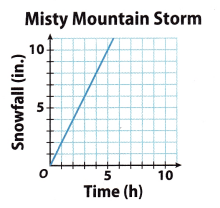A. Find the slope of the graph using the points (1, 2) and (5, 10). Remember that the slope is the constant rate of change.

B. Find the unit rate of snowfall in inches per hour. Explain your method.

C. Compare the slope of the graph and the unit rate of change in the snow level. What do you notice?

D. Which point on the graph tells you the slope of the graph and the unit rate of change in the snow level? Explain how you found the point.

Reflect

Question 1.
What If? Without referring to the graph, how do you know that the point (1, $$\frac{4}{3}$$) is on the graph?
Lets find the slope:
Slope = $$\frac{8-4}{6-3}$$ = $$\frac{4}{3}$$
This holds for every two points on the graph, so it has to hold for some of the given points and the point we want to check. Substitute the point (6, 8) for (1, $$\frac{4}{3}$$) and see if it still holds.
$$\frac{\frac{4}{3}-4}{1-3}$$ = $$\frac{4}{3}$$ = Slope
for x = Time and y = Amount
Therefore, the point (1, $$\frac{4}{3}$$) is on the line.
By checking if the equation for the slope (which we calculated) holds for the given point we see that it does, so it is on the line.

Question 2.
Tomas rides his bike at a steady rate of 2 miles every 10 minutes. Graph the situation. Find the unit rate of this proportional relationship.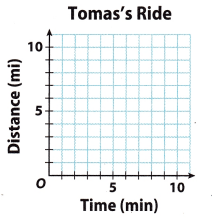Make a table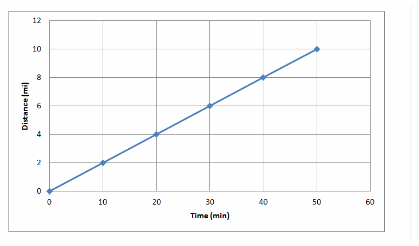Graph the solution
slope = $$\frac{\text { Rise }}{\text { Run }}$$ = $$\frac{4-2}{20-10}$$ = $$\frac{2}{10}$$ = $$\frac{1}{5}$$ Find the slope
The unit rate and slope of a graph of the ride is $$\frac{1}{5}$$ mi per min

Reflect

Question 3.
Describe the relationships among the slope of the graph of Well A’s rate, the equation representing Well A’s rate, and the constant of proportionality.
The slope of the graph of Well, A’s rate, the equation representing Well A’s rate and the constant of proportionality are the same. They all represent the amount of oil pumped from Well A in an hour.

Question 4.
The equation y = 375x represents the relationship between x, the time that a plane flies in hours, and y, the distance the plane flies in miles for Plane A. The table represents the relationship for Plane B. Find the slope of the graph for each plane and the plane’s rate of speed. Determine which plane is flying at a faster rate of speed.Notice that for every value of x and y for the Plane A the given equation has to hold. So
y = 375x
therefore
k = $$\frac{y}{x}$$ = 375 is the slope of the graph for Plane A.
Using the table we can find the slope of the graph for Plane B:
$$\frac{y_{2}-y_{1}}{x_{2}-x_{1}}$$ = $$\frac{850-425}{2-1}$$ = 425
Therefore, the slope of the graph of Plane B is greater than the slope of the graph of Plane A. We can conclude that the Plane B is faster because it flies more miles per hour (faster rate of speed).
Plane B is flying at the faster rate of speed.

Give the slope of the graph and the unit rate. (Explore Activity and Example 1)

Question 1.
Jorge: 5 miles every 6 hours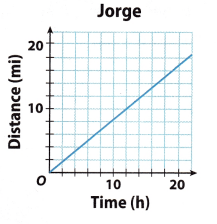we are given that Jorge travels 5 miles every 6 hours. ‘Therefore, we construct the table and draw a graph:slope = $$\frac{\text { rise }}{\text { run }}$$ = $$\frac{5}{6}$$
The unit of the distance travelled and the slope of the graph of the relationship are equal, $$\frac{5}{6}$$ miles per hour.
$$\frac{5}{6}$$ miles per hour.

Question 2.
Akiko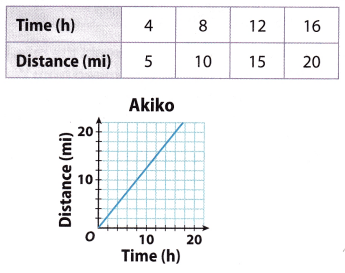slope = $$\frac{\text { Rise }}{\text { Run }}$$ = $$\frac{10-5}{8-4}$$ = $$\frac{5}{4}$$
The unit rate is $$\frac{5}{4}$$ miles per hour.
Slope: $$\frac{5}{4}$$, unit rate: $$\frac{5}{4}$$ miles per hour

Question 3.
The equation y = 0.5x represents the distance Henry hikes in miles over time in hours. The graph represents the rate that Clark hikes. Determine which hiker is faster. Explain. (Example 2)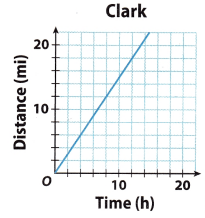y = 0.5x Equation representing Henry’s rate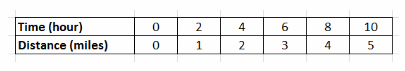Use the equation to make a table
Slope = Unit rate = $$\frac{2-1}{4-2}$$ = $$\frac{1}{2}$$ miles per hour
Use the table to find the slope of the graph of Plane A
Slope = Unit rate = $$\frac{18-6}{12-4}$$ = $$\frac{12}{8}$$ miles per hour
Use the graph to find the slope of the graph of Clark
Clark is faster Compare the units
$$\frac{3}{2}$$ > $$\frac{1}{2}$$

Write an equation relating the variables in each table. (Example 2)

Question 4.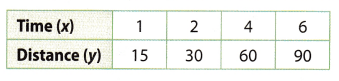Fmd the slope and with it the unit rate:
Slope = $$\frac{30-15}{2-1}$$ = 15 = Unit rate
Now we know that for every x and y the following equation holds:
$$\frac{y}{x}$$ = 15
therefore
y = 15x

Question 5.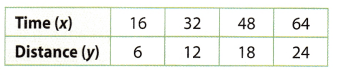Slope = Unit rate = $$\frac{12-6}{32-16}$$ = $$\frac{6}{16}$$ = $$\frac{3}{8}$$ Find the slope using the table
y = $$\frac{3}{8}$$x Writing the equation

Essential Question Check-In

Question 6.
Describe methods you can use to show a proportional relationship between two variables, x and y. For each method, explain how you can find the unit rate and the slope.
Explanation A:
We can represent a proportional relationship between two variables x and y using a table. For each point we find
the ratio of y over x. If this ratio is constant over each point, then the relationship is proportional. To find the unit rate and slope, we have:
Slope = Unit Rate = $$\frac{y_{2}-y_{1}}{x_{2}-x_{1}}$$

We can represent a proportional relationship between two variables x and y using an equation of the form y = kx, where k is a number called the constant of proportionality. The slope and unit rate are equal to the constant of proportionality, however we can construct a table from the given equation and proceed as described above.

We can represent a proportional relationship between two variables x and y using a graph. The graph will be a line that passes through the origin (0, 0). To find the unit rate and slope, we have:
Slope = Unit Rate = $$\frac{\text { rise }}{\text { run }}$$

Explanation B:
You can use a table by finding the ratio of each point. If the ratio is constant, the relationship is proportional. You
can find the unit rate and slope by:
Slope(m) = UnitRate = $$\frac{y_{2}-y_{1}}{x_{2}-x_{1}}$$

You can use a graph by finding ratio of the rise for equal interval of run. You can find the unit rate and slope by:
Slope(m) = UnitRate = $$\frac{\text { rise }}{\text { run }}$$

Question 7.
A Canadian goose migrated at a steady rate of 3 miles every 4 minutes.
a. Fill in the table to describe the relationship.Top row: 12, 16; Bottom row: 3, 6, 15

b. Graph the relationship.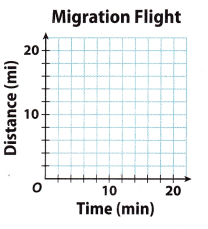Click for graph.

c. Find the slope of the graph and describe what it means in the context of this problem.
The slope of the graph is
$$\frac{6-3}{8-4}$$ = $$\frac{3}{4}$$
in this case this means that a Canadian goose migrates 3 miles every 4 minutes or $$\frac{3}{4}$$ miles every minute.
The goose migrates $$\frac{3}{4}$$ miles every minute.

Question 8.
Vocabulary A unit rate is a rate in which thein the comparison is one unit.
A unit is a rate in which the second quantity in the comparison is one unit.

Question 9.
The table and the graph represent the rate at which two machines are bottling milk in gallons per second.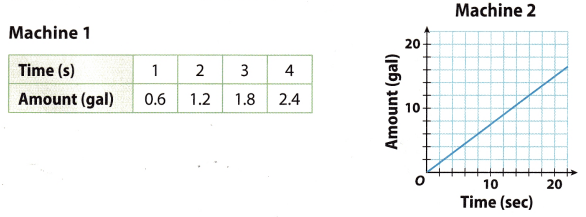a. Determine the slope and unit rate of each machine
(1) Using the given table, calculate the slope and unit rate of the first machine.
Slope = Unit rate = $$\frac{1.2-0.6}{2-1}$$ = $$\frac{0.6}{1}$$ = 0.6
The unit rate for the first machine is therefore 0.6 gallons per second.

(2) Using the given graph, find points to substitute into the formula for the slope.
Two points on the line for the second machine are: (8, 6) and (16, 12).
Now calculate the slope and unit rate of the second machine using the points you just found.
Slope = Unit rate = $$\frac{12-6}{16-8}$$ = $$\frac{6}{8}$$ = 0.75
The unit rate for the second machine is therefore 0.75 gallons per second
The slope and unit rate of the first machine is 0.6 (gallons per second), and of the second 0.75 (gallons per
second).

b. Determine which machine is working at a faster rate.
Comparing the unit rates of both machines we can see that the second machine bottles more milk per second than the first machine. Therefore it is working at a faster rate.
The second machine is faster.

Question 10.
Cycling The equation y = $$\frac{1}{9}$$x represents the distance y, in kilometers, that Patrick traveled in x minutes while training for the cycling portion of a triathlon. The table shows the distance y Jennifer traveled in x minutes in her training. Who has the faster training rate?From y = $$\frac{1}{9}$$x we can make a table for Patrick’s unit rate in kilometers per minute.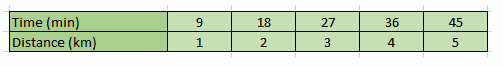Now,
$$\frac{2-1}{18-9}$$ = $$\frac{1}{9}$$
Therefore, Patrick’s unit rate is $$\frac{1}{9}$$ kilometers per minute.

Lets find the slope and the unit rate of jennifer
Slope = $$\frac{8-5}{64-40}$$ = $$\frac{3}{24}$$ = $$\frac{1}{8}$$
Therefore, Jennifer’s unit rate is $$\frac{1}{8}$$ kilometers per minute.
We can conclude that Patrick has a faster training rate.

Question 11.
Analyze Relationships There is a proportional relationship between minutes and cost in dollars. The graph passes through the point (1, 4.75). What is the slope of the graph? What is the unit rate? Explain.
If we know that there is a proportional relationship between minutes and dollars per minute, then the graph is a
straight line. Also since that line passes through (1, 4.75) we know that for 1 minute it costs 4.75 dollars to print.
Therefore the slope is 4.75 and the unit rate is 4.75 dollars per minute.

The slope is 4.75 and the unit rate is 4.75 dollars per minute.

Question 12.
Draw Conclusions Two cars start at the same time and travel at different constant rates. The graph of the distance in miles given the time in hours for Car A passes through the point (0.5, 27.5), and the graph for Car B passes through the point (4, 240). Which car is traveling faster? Explain.
Unit Rate = $$\frac{27.5}{0.5}$$ = 55 Find the unit rate for Car A.
Car $A$ travels $55$ miles in $1$ hr

Unit Rate = $$\frac{240}{4}$$ = 60 Find the unit rate for Car B.
Car $B$ travels $60$ miles in $1$ hr
Car B is faster Compare the unit rates
60 > 55

Question 13.
Critical Thinking The table shows the rate at which water is being pumped into a swimming pool.Use the unit rate and the amount of water pumped after 12 minutes to find how much water will have been pumped into the pool after 13$$\frac{1}{2}$$ minutes. Explain your reasoning.
Unit rate = $$\frac{216-36}{12-2}$$ = $$\frac{180}{10}$$ = 18 Find the unit rate
Additional water = 18 * 1$$\frac{1}{2}$$ = 27 After 1$$\frac{1}{2}$$ min after 12 min
Total = 216 + 27 = 243gal The total water after 13$$\frac{1}{2}$$ min